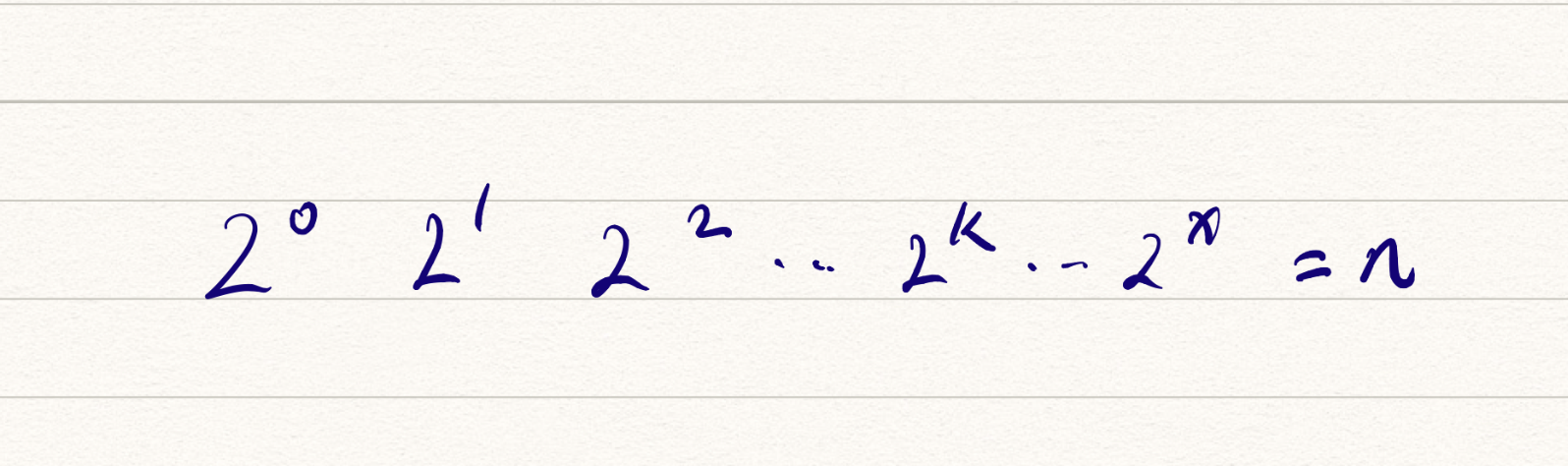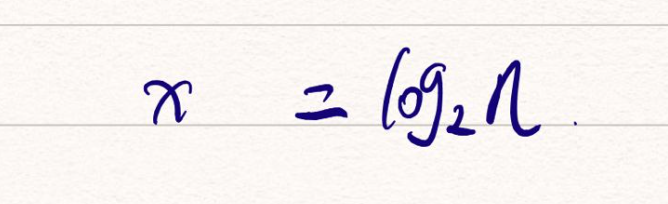### 为什么需要分析复杂度？

• 测试结果依赖测试环境
• 测试结果受规模数据影响很大

#### 1. 测试结果非常依赖测试环境

【那控制变量不就好了？将 a,b 都跑在一样的机器上，但是有种情况就是数据量不大的时候，算法效率差距不大，当数据量极大时，算法差距会呈指数形式，所以我们需要的不是具体的执行时间，是一种抽象的效率表达式

#### 2. 测试结果受数据规模影响很大

• 小规模的数据排序，插入排序可能比快排更快。

### 大 O 复杂度表示法

int cal(int n) {
int sum = 0;
int i = l;
for(; i<= n; i++) {
sum = sum + i;
}
return sum;
}


2、3行分别需要 1unit_time 执行时间，4、5 行运行了 n 遍，需要 2n * unit_time 的执行时间，所以总执行(2n + 2) * unit_time 个运行时间，所有代码的运行时间 T(n) 与每行代码的执行次数成正比

int cal(int n) {
int sum = 0;
int i = 1;
int j = 1;
for (; i <= n; ++i) {
j = 1;
for (; j <= n; ++j) {
sum = sum + i * j;
}
}
}


• 2、3、4 行尾赋值语句，各需要 1 个 unit_time
• 5、6 行循环了 n 遍，需要 2n * unit_time
• 7、8行执行了 n^2 遍，需要 2n^2 * unit_time 执行时间

T(n) = O(f(n)) ，下面是这个表达式的详解：

• T(n) --> 代码执行时间

• n --> 数据规模大小

• f(n) --> 每行代码执行的次数总和

• O --> 在上个表达式中表示 代码执行时间 T(n) 与 f(n) 成正比

n 很大时，可以想象成 10,00010,000,000，公式中的 低阶、常量、系数不左右增长趋势，可以忽略。我们只需要记录 最大量级，用 大O 表示法表示刚才两端代码的复杂度，分别记录为：

• T(n) = O(n)
• T(n) = O(n^2)

### 时间复杂度分析

#### 1. 只关注循环执行次数最多的一段代码

int cal(int n) {
int sum = 0;
int i = 1;
for (; i <= n; ++i) {
sum = sum + 1;
}
return sum;
}

• 2、3行执行赋值操作，耗费的是常量的执行时间，与数据规模 n 无关，对于复杂度分析没有影响
• 循环执行次数最多的代码是 4、5for 循环代码，所以这块需要重点分析，这两行代码被循环了 n 次，所以总时间复杂度是 O(n)

#### 2.加法法则：总复杂度等于量级最大的那段代码的复杂度

int cal(int n) {
int sum_1 = 0;
int p = 1;
for (; p < 100; ++p) {
sum_1 = sum_1 + p;
}

int sum_2 = 0;
int q = 1;
for (; q < n; ++q) {
sum_2 = sum_2 + q;
}

int sum_3 = 0;
int i = 1;
int j = 1;
for (; i <= n; ++i) {
j = 1;
for (; j <= n; ++j) {
sum_3 = sum_3 + i * j;
}
}
return sum_1 + sum_2 + sum_3;
}


• 第一段复杂度是常数：100，所以直接忽略

• 第二段是 O(n) 复杂度

• 第三段循环是复杂度量级最大的循环，所以直接分析第三段循环即可，第三段做了 for 循环嵌套，每次的复杂度是 O(n) ，所以总复杂度是 O(n^2)

• 算法执行效率与数据规模增长的变化趋势，所以常量可以忽略

#### 3.乘法法则：嵌套代码的复杂度等于嵌套内外代码复杂度的乘积

• T1(n) = O(f(n))
• T2(n) = O(g(n))
• *T(n) = T1(n) * T2(n) = O(f(n)) * O(g(n)) = O(f(n)g(n))

// 这里调用了另外一个函数 f()，所以可以看做是循环
int cal(int n) {
int ret = 0;
int i =1;
// for 循环本身复杂度为 O(n) ，循环中调用了 复杂度为 O(n) 的 f()
// 所以最终 cal() 的复杂度为 O(n^2)
for (; i < n; ++i) {
ret = ret + f(i);
}
}

// 复杂度为 O(n)
int f(int n) {
int sum = 0;
int i =1;
for (; i <= n; ++i) {
sum = sum + i;
}
return sum;
}


【作者的分析和我的相同，我的分析已经使用注释的方式写在上面的代码中了。】

### 几种常见的复杂度分析实例

• 常量级 —— O(1)
• 指数级 —— O(2^n)
• 对数级 —— O(log(n))
• 线性级 —— O(n)
• 线性对数 —— O(nlogn)
• 平方级 —— O(n^2)
• 立方级 —— O(n^3)
• k次方级 —— O(n^k)
• 阶乘级 —— O(n!)

• 指数级 —— O(2^n)
• 阶乘级 —— O(n!)

#### 1. O(1)

int i = 8;
int j = 6;
int sum = i + j;


【反过来说，尽量避免循环和递归的存在。】

#### 2. O(logn)、O(nlogn)

i =  1;
while (i <= n) {
i = i * 2;
}i = 1;
whiel (i <= n) {
i = i * 3;
}#### 3. O(m+n)、O(m*n)

int cal(int m, int n) {
int sum_1 = 0;
int i  = 1;
for (; i < m; ++i) {
sum_1 = sum_1 + i;
}

int sum_2 = 0;
int j = 1;
for (; j < n; ++j) {
sum_2 = sum_2 + j;
}

return sum_1 + sum_2;
}


T1(m) + T2(n) = O(f(m) + g(n))

T1(m) * T2(n) = O(f(m) * f(n))

### 空间复杂度分析

void print(int n) {
int i = 0;
int[] a = new int[n];
for (i; i < n; ++i) {
a[i] = i * i;
}
for (i = n-1; i >= 0; --i) {
print out a[i];
}
}


• 第 2 行代码申请了一个空间存储变量 i，但是是常量级，所以可以忽略。
• 第3行申请了一个大小为 nint 类型数组，除此之外，代码没有占用更多的空间，所以这段代码的空间复杂度是 O(n)

• O(1)
• O(n)
• O(n^2)

• O(1)
• O(logn)
• O(n)
• O(nlogn)
• O(n^2)

Q.E.D.